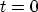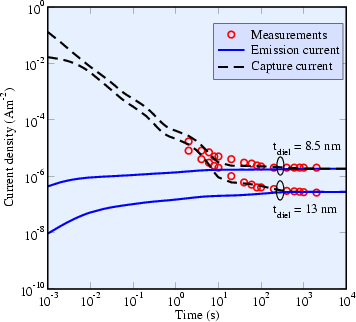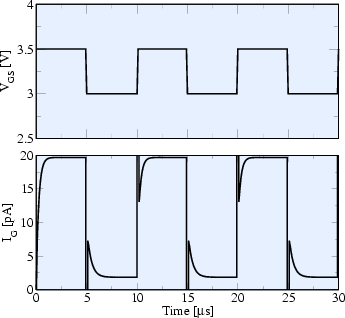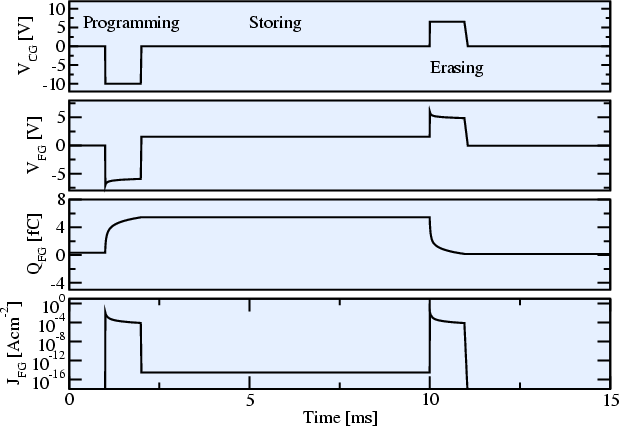### 5.2.1.2 Transient SILC in EEPROMs

It has been shown that the transient trap-assisted tunneling current can be described by a rate equation which gives rise to an exponential behavior of the tunneling current over time, see Section 3.8.2.4. The left part of Fig. 5.27 shows measurements of the gate current density of MOS capacitors as a function of time with dielectric thicknesses of 8.5nm and 13.0nm, compared to simulations . Initially, the traps are empty which can be achieved by applying flat band conditions. Ats, the gate voltage is turned on (-5.8V and -8.3V for the thinner and the thicker dielectric, respectively) and the traps are filled according to their specific capture and emission time constants. This charging current consists of an emission and a capture current, which may exceed the steady-state current by orders of magnitude. A good fit to the measured data can be achieved using the trap parameters indicated in the figure caption.

The right part of Fig. 5.27 shows the gate current of an MOS capacitor for an applied rectangular pulse with a frequency of 100kHz assuming initial flat band conditions. It can be seen that the time constants of the trap filling and emptying processes are not equal but depend on the applied voltage, since different voltages lead to different capture and emission times. The spikes in this figure are due to the sudden voltage change while the trap concentration remains constant: In the transition from 3.0V to 3.5V the barrier shape changes suddenly, and traps are rapidly emptied. Traps near the cathode are filled and it takes several micro seconds until the new steady state is reached. Thus, dielectric materials which have such a high trap concentration may lead to considerable problems for high-frequency applications.For EEPROM devices the charging and discharging characteristics are crucial: Programming and erasing should happen as fast as possible, therefore, high voltages are applied. The discharging current over time, on the other hand, determines the retention time and must be very low. To allow the simulation of these characteristics, the contact condition Floating was implemented in MINIMOS-NT. For floating contacts, the electrostatics in the device is acquired in the initial time step. Then, the current contact condition at the floating gate contact is set to zero (no out-flowing contact current) . The tunneling current to or from the floating gate changes the charge and thus the voltage on the contact. For the simulation of the programming or erasing processes, first all voltages are set to zero (the voltage which is assumed to represent the empty state). Then, the charge at the floating gate is used as charge contact condition and the programming voltage is applied at the control gate. Fig. 5.28 shows the control gate voltage, floating gate voltage, floating gate charge, and tunneling current for the programming, storing, and erasing processes. It can be seen that the programming and erasing pulses must be carefully optimized to avoid over-erase, since the tunnel current density for positive and negative voltages on the floating gate is not equal. This is frequently addressed in the literature [278,279].A. Gehring: Simulation of Tunneling in Semiconductor Devices